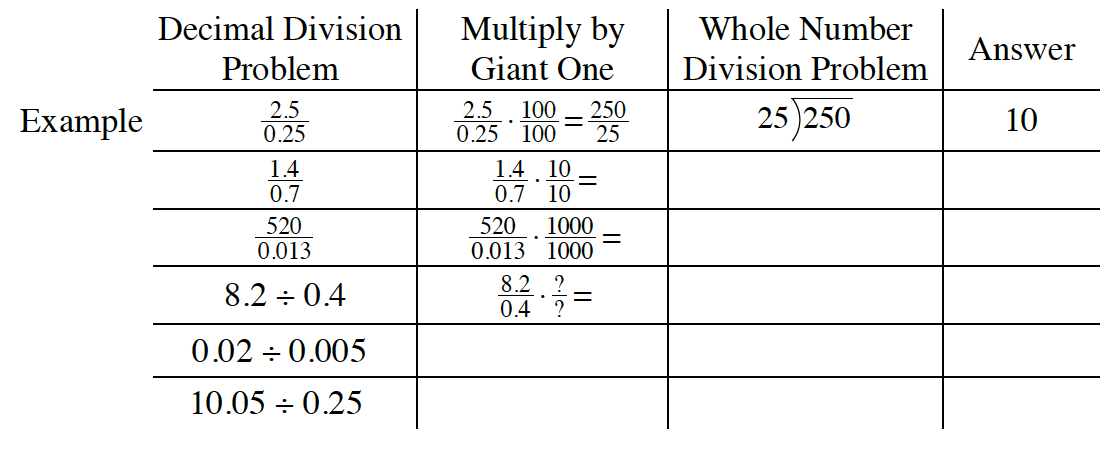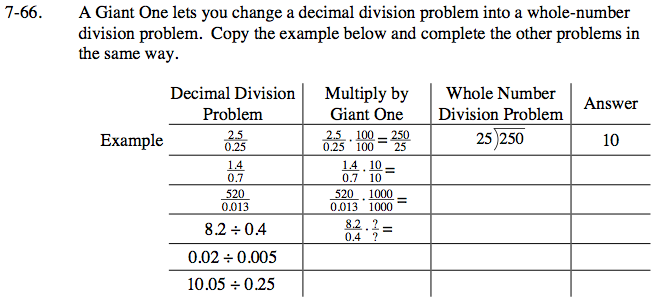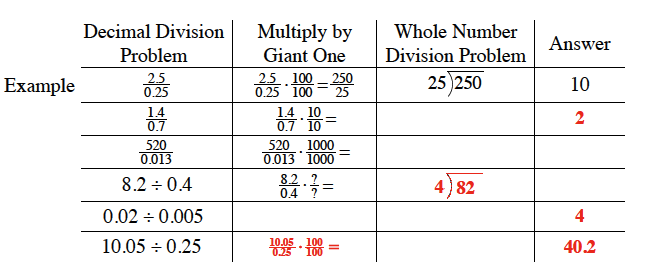### Home > CC1 > Chapter 7 > Lesson 7.2.3 > Problem7-66

7-66.
1. A Giant One lets you change a decimal division problem into a whole-number division problem. Copy the example below and complete the other problems in the same way. Homework Help ✎

2.Follow the given example and multiply by a Giant One to move the decimal points the necessary places to make whole numbers.
Keep in mind that the number of places behind the decimal point in the denominator is equivalent to the number of zeros needed in your Giant One.

Notice that there are 4 numbers to the right of the decimal point and that there are also 4 zeros in the numerator and denominator of your Giant One.
Now try filling in the rest of the above chart by rewriting the fraction as a whole number division problem and solving the division problem.